# Determine surge and swab pressure for open-ended pipe

You’ve learn how to determine surge and swab pressure from the previous topic (Surge and swab pressure method 1) and this topic will demonstrate you how to use those equations to determine surge and swab pressure for the open-ended pipe.

Example

The well information is listed below:

θ300 = 85

θ600 = 130

Hole diameter = 9 inch

Drill Collar = 6.25 inch

ID of drill collar = 2.5 inch

Drillpipe diameter = 5 inch

ID of drill pipe = 3.5 inch

Average pipe running or pulling speed = 250 ft/min

Drill Pipe Length = 12000 ft

Drill Collar Length = 800 ft

Current Mud Weight = 12.5 ppg

Well TVD = 9000 ft

## 1st case – open ended pipe

Determine pressure loss around drill pipe

1. Determine n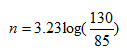n= 0.613

2. Determine K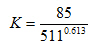K=1.863

3. Determine fluid velocity around drill pipe

For open-ended pipe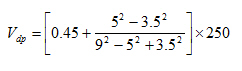Vdp = 159.2 ft/min

4. Maximum pipe velocity

Vm = 159.2 x 1.5 = 239 ft/min

5. Pressure loss around drill pipe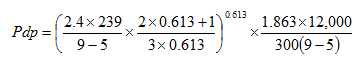Pdp =438.5 psi

Determine pressure loss around drill collar

We still use the same n and K.

1. Determine fluid velocity around drill collar

For open ended pipe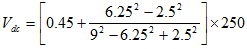Vdc = 282.7 ft/min

2. Maximum pipe velocity

Vm = 282.7 x 1.5 = 424 ft/min

3. Pressure loss around drill collar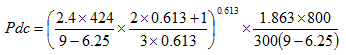Pdc = 76.1 psi

Total pressure loss = Pdp + Pdc

Total pressure loss = 438.5 + 76.1 = 514.6 psi

If surge pressure is required: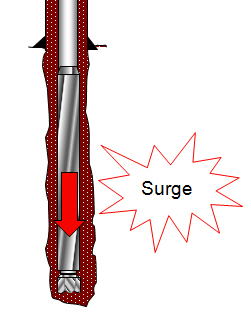Surge pressure = hydrostatic pressure + total pressure loss

Surge pressure = (0.052 x 9000 x 12.5) + 514.6 = 6364.6 psi

If swab pressure is required: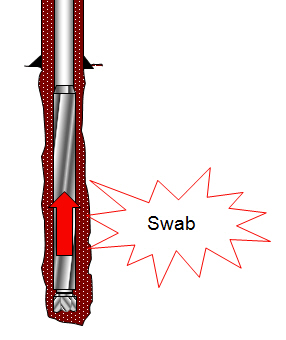Swab pressure = hydrostatic pressure – total pressure loss

Swab pressure = (0.052 x 9000 x 12.5) – 514.6 = 5335.4 psi

Share the joy
Tagged , , , . Bookmark the permalink.